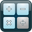»  » Online Calculator# Online Calculator

## How to use Online Calculator

This is a calculator that will help you to do the complex calculations like extracting the roots, trigonometric functions, logarithms, percentages, etc. Just use it like the standard calculator click on the numbers and tasks you want it to calculate for you. When you press any task button like division, subtracting, the white ring may appear to show you that an operation can be done by this tool. The calculator uses the package of algebraic plugins that allows you to type the sequence in the same order it stated originally. You can also choose a “simple” mode of this online calculation tool if you just need to perform simple algebraic calculations.

• Simple
0
2nd
(
)
%
1/x
x2
x3
yx
x!
xy
log
sin
cos
tan
ln
sinh
cosh
tanh
ex
Deg
π
EE
Rand
mc
m+
m-
mr
AC
+/–
÷
×
7
8
9
4
5
6
+
1
2
3
=
0
.